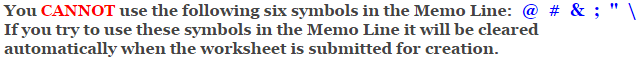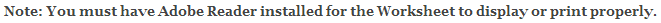# Fractions Worksheets

## Subtracting Fractions and Whole Numbers Worksheets

This fraction worksheet is for practicing subtracting fractions from whole numbers. You can select from five different degrees of difficulty. The easiest will keep the denominators and the numerators between 1 and 9. The hardest will keep the numerators between 1 and 20. The answer worksheet will show the progression on how to solve the problems. First convert the whole number to a fraction. Then subtract and check to see if we need to simplify or reduce the mixed number. This fraction worksheet will generate 10 or 15 mixed number subtraction problems per worksheet.

### Difficulty

Numerators 1 thru 9.
Denominators 1 thru 12.

Numerators 1 thru 9.
Denominators 2, 3, 4, 5, & 10.

Numerators 1 thru 12.
Denominators up to 30.

Numerators 1 thru 16.
Denominators up to 60.

Numerators 1 thru 20.
Denominators up to 120.

### Language for the Fractions Worksheet

 English German Albanian Spanish Swedish Italian French Turkish Polish Norwegian

### Memo Line for the Fractions Worksheet

You may enter a message or special instruction that will appear on the bottom left corner of the Fractions Worksheet.Now you are ready to create your Fractions Worksheet by pressing the Create Button.

 Recommended Videos

If You Experience Display Problems with Your Math Worksheet